Next: 3.2.2 The Corrected Asymmetry Up: 3.2 Measuring the Internal Previous: 3.2 Measuring the Internal

## 3.2.1 The Raw Asymmetry for a Pair of Counters

Consider a pair of opposing counters--e.g. L and R in Fig. 3.1 with a magnetic field applied parallel to the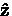axis. The raw asymmetry'' of the histograms NL (t) and NR (t) is defined as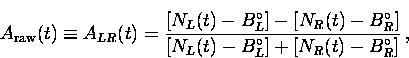(7)

where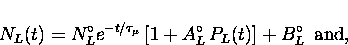(8)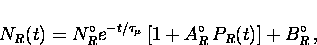(9)

so that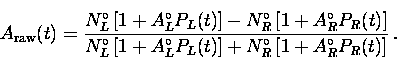(10)

The reason for introducing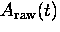is to eliminate the muon lifetime (which is a well known quantity) and the random backgrounds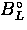and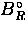. Ideally, the two counters in question are identical to one another, so that the histograms recorded by the two counters differ only by a phase. In this idealistic situation the difference in phase between the histograms is due solely to the geometry of the positron counters with respect to the sample. The polarization of the muon spin which is seen by each counter is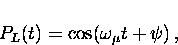(11)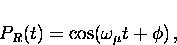(12)
where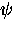and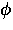are the initial phases of the muon spin polarization vector in counters L and R, respectively. If the counters are aligned precisely opposite one another, the difference between these phases is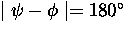, so that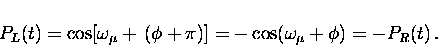(13)
The counters L and R measure the projection of the muon polarization on the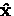axis so that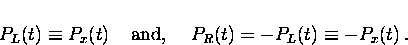(14)
Thus Eq. (3.7) becomes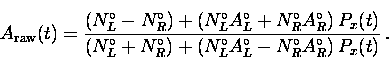(15)
If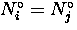and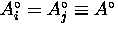then Eq. (3.15) reduces to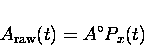(16)
If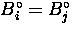, then using Eqs. (3.7), (3.8), (3.9) and (3.16) the x-component of the polarization can be written as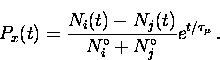(17)Next: 3.2.2 The Corrected Asymmetry Up: 3.2 Measuring the Internal Previous: 3.2 Measuring the Internal